# Samacheer Kalvi 12th Maths Solutions Chapter 7 Applications of Differential Calculus Ex 7.1

## Tamilnadu Samacheer Kalvi 12th Maths Solutions Chapter 7 Applications of Differential Calculus Ex 7.1

Question 1.
A point moves along a straight line in such a way that after t seconds its distance from the origin is s = 2t2 + 3t metres.
(i) Find the average velocity of the points between t = 3 and t = 6 seconds.
(ii) Find the instantaneous velocities at t = 3 and t = 6 seconds.
Solution:
(i) Given s = 2t2 + 3t
(i.e.) f(t) = 2t2 +3t
Now f(3) = 18 + 9 = 27 = f(a)
f(6) = 72 + 18 = 90 = f(b)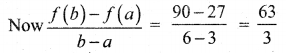= 21m/s

(ii) f(t) = 2t2 + 3t
f'(t) = 4t + 3
f'(3) = 4(3) + 3 = 15
f'(6) = 4(3) + 3 = 15

Question 2.
A camera is accidentally knocked off an edge of a cliff 400 ft high. The camera falls a distance of s = 16t2 in t seconds.
(i) How long does the camera fall before it hits the ground?
(ii) What is the average velocity with which the camera falls during the last 2 seconds?
(iii) What is the instantaneous velocity of the camera when it hits the ground?
Solution:
(i) s = 16t2
16t2 = 400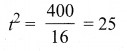t = 5sec(ii) Last 2 seconds means t = 3 to t = 5
f(t) = 16t2
f(3) = 16(9) = 144 = f(a)
f(5) = 16(25) = 400 = f(b)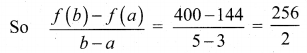= 128 ft/sec

(iii) f(t) = 2
f'(t) = 32t
f'(t) at t = 5 = 32(5)
= 160 ft/sec

Question 3.
A particle moves along a line according to the law s(t) = 2t3 – 9t2 + 12t – 4, where t ≥ 0.
(i) At what times the particle changes direction?
(ii) Find the total distance travelled by the particle in the first 4 seconds.
(iii) Find the particle’s acceleration each time the velocity is zero.
Solution:
(i) s = f(t) = 2t3 – 9t2 + 12t – 4
V = f'(t) = 6t2 – 18t + 12
V = 0 ⇒ 6(t2 -3t – 2) = 0
(t – 1)(t – 2) = 0
t = 1, 2
When t < 1, (say t = 0.5)
V = 6(0.25 – 1.5 + 2) = +ve
When 1 < t < 2, (say t = 1.5) V = 6(2.25 – 4.5 + 2) = -ve When t > 2, (say t = 3)
V = 6(9 – 6 + 2) = +ve
So the particle changes its direction when t lies between 1 and 2 secs.

(ii) The distance travelled in the first 4 seconds is
|s(0) – s(1)| + |s(1) – s(2)| + |s(2) – s(3)| + |s(3) – s(4)|
Here, s(t) = 2t3 – 9t2 + 12t – 4
s(0) = -4
s(1) = 1
s(2) = 0
s(3) = 5
and s(4) = 28
∴ Distance travelled in first 4 seconds
= |-4 – 1| + |1 – 0| + |0 – 5| + |5 – 28|
= 5 + 1 + 5 + 23 = 34 m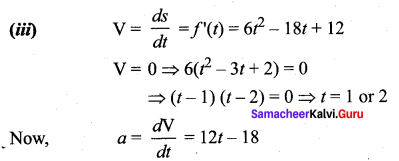a (at V = 0) is ‘a’ at t = 1 and 2
Now a (at t = 1) = 12 – 18 = -6m / sec2
a (at t = 2) = 24 – 18 = +6m / sec2

Question 4.
If the volume of a cube of side length x is V = x3. Find the rate of change of the volume with respect to x when x = 5 units.
Solution: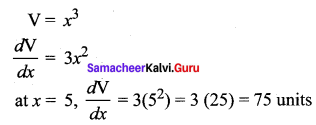Question 5.
If the mass m(x) (in kilograms) of a thin rod of length x (in metres) is given by, m(x) = $$\sqrt{3 x}$$ then what is the rate of change of mass with respect to the length when it is x = 3 and x = 21 metres.
Solution: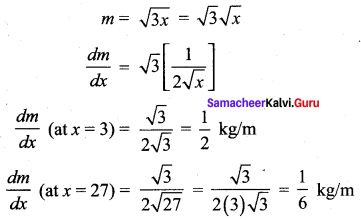Question 6.
A stone is dropped into a pond causing ripples in the form of concentric circles. The radius r of the outer ripple is increasing at a constant rate at 2 cm per second. When the radius is 5 cm find the rate of changing of the total area of the disturbed water?
Solution: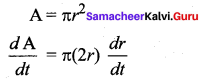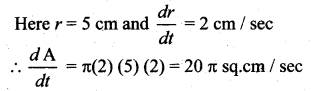Question 7.
A beacon makes one revolution every 10 seconds. It is located on a ship which is anchored 5 km from a straight shore line. How fast is the beam moving along the shore line when it makes an angle of 45° with the shore?
Solution:
Time for 1 rotation = 10 sec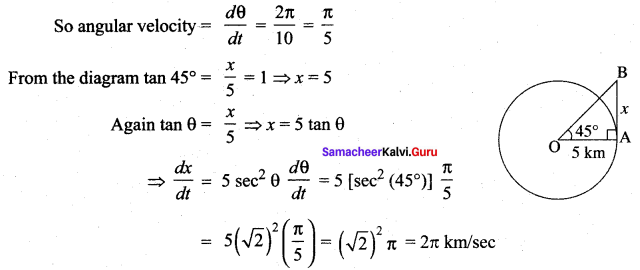Question 8.
A conical water tank with vertex down of 12 metres height.has a radius of 5 metres at the top. If water flows into the tank at a rate 10 cubic m/min, how fast is the depth of the water increases when the water is 8 metres deep?
Solution: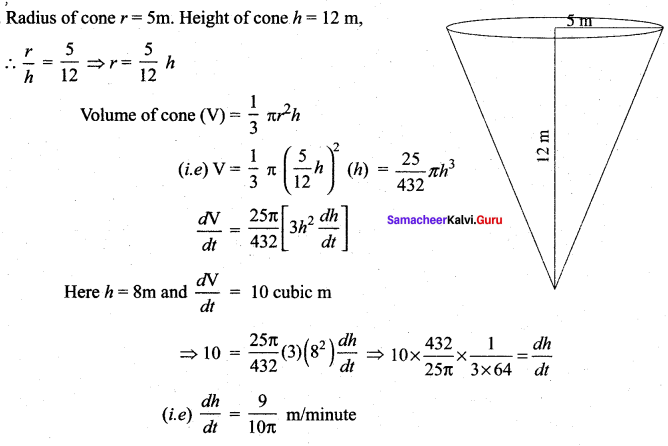Question 9.
A ladder 17 metre long is leaning against the wall. The base of the ladder is pulled away from the Waif at a rate of 5 m/s. When the base of the ladder is 8 metres from the wall
(i) How fast is the top of the ladder moving down the wall?
(ii) At what rate, the area of the triangle formed by the ladder, wall, and the floor, is changing?
Solution: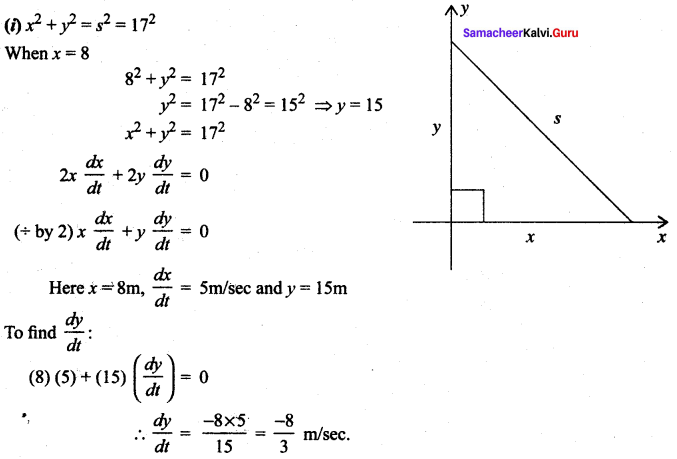So the top of the ladder is moving down the wall at $$\frac{-8}{3}$$ m/sec

(ii)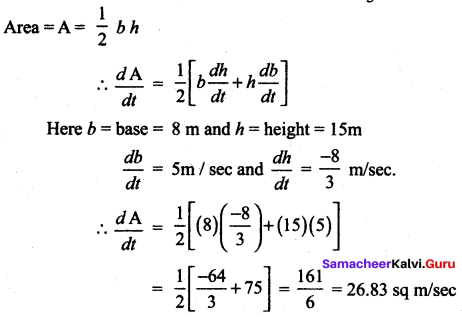Question 10.
A police jeep, approaching an orthogonal intersection from the northern direction, is chasing a speeding car that has turned and moving straight east. When the jeep is 0.6 km north of the intersection and the car is 0.8 km to the east. The police determine with a radar that the distance between them and the car is increasing at 20 km/hr. If the jeep is moving at 60 km/hr at the instant of measurement, what is the speed of the car?
Solution: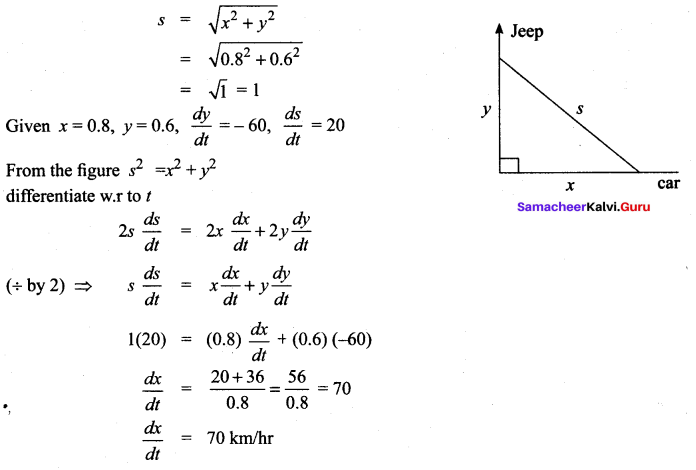### Samacheer Kalvi 12th Maths Solutions Chapter 7 Applications of Differential Calculus Ex 7.1 Additional Questions

Question 1.
A water tank has the shape of an inverted circular cone with base radius 2 metres and height 4 metres. If water is being pumped into the tank at a rate of 2mt3/min, find the rate at which the water level is rising when the water is 3m deep.
Solution:
We first sketch the cone and label it as in diagram. Let V, r and h be respectively the volume of the water, the radius of the cone and the height at time t, where t is measured in minutes.
We are given that $$\frac{d \mathrm{V}}{d t}$$ = 2m3/min and we are asked to find $$\frac{d h}{d t}$$ when h is 3m.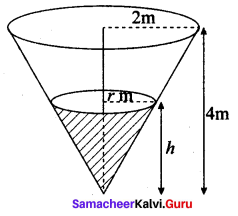The quantities V and h are related by the equation V = $$\frac{1}{3} \pi r^{2} h$$. But it is very useful to express V as function of h alone.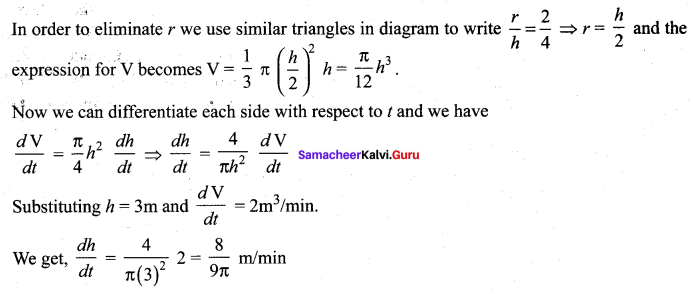Question 2.
A car A is travelling from west at 50 km/hr and car B is travelling towards north at 60 km/hr. Both are headed for the intersection of the two roads. At what rate are the cars approaching each other when car A is 0.3 kilometers and car B is 0.4 kilometers from the intersection?
Solution:
We draw diagram where C is the intersection of the two roads. At a given time t, let x be the distance from car A to C, let y be the distance from car B to C and let z be the distance between the cars A and B where x, y and z are measured in kilometers.
We are given that $$\frac{d x}{d t}$$ = – 50 km/hr and $$\frac{d y}{d t}$$ – = -60 km/hr.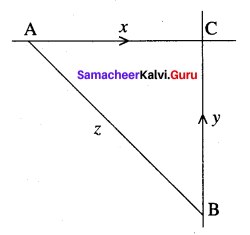Note that x andy are decreasing and hence the negative sign.
We are asked to find $$\frac{d z}{d t}$$
The equation that relate x, y and z is given by the pythagoras theorem z2 = x2 + y2
Differentiating each side with respect to t,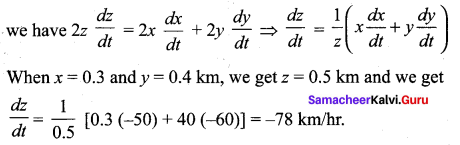i.e., The cars approaching each other at a rate of 78 km/hrQuestion 3.
The distance x metres travelled by a vehicle in time t seconds after the brakes are applied is given by x = 20t – $$\frac{5}{3}$$t2. Determine
(i) the speed of the vehicle (in km/hr) at the instant the brakes are applied and
(ii) the distance the car travelled before it stops.
Solution: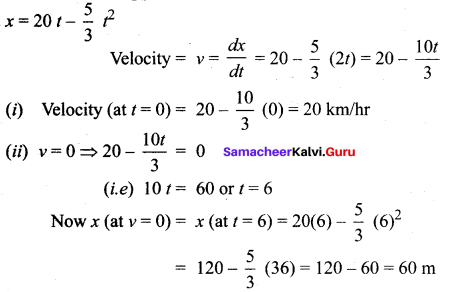Question 4.
At noon, ship A is 100 km west of ship B. Ship A is sailing east at 35 km/hr and ship B is sailing north at 25 km/hr. How fast is the distance between the ships changing at 4.00 pm?
Solution:
Distance travelled by A in 4 hrs
(12 noon to 4.00 pm) = 4 × 35 = 140 km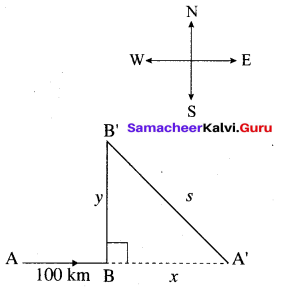∴ BA’= 40 km (140 – 100)
Distance travelled by B in 4 hrs = 4 × 25 = 100 km
Now in right angled ∆A’BB’, x2 + y2 = s2
Here, x = BA’ = 40 km
y = BB’= 100 km
⇒ s2 = 402 + 1002
= 1600 + 10000
= 11600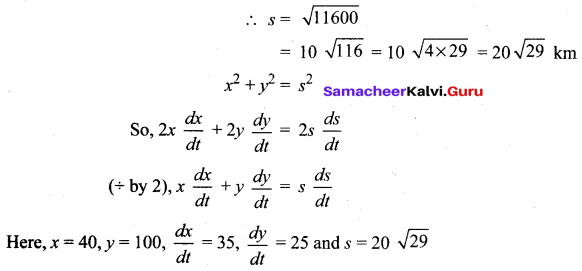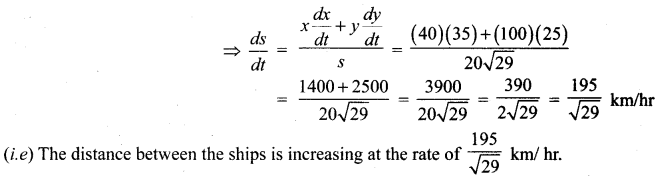Question 5.
Gravel is being dumped from a conveyor belt at a rate of 30 ft3/min and its coarsened such that it forms a pile in the shape of a cone whose base diameter and height are always equal. How fast is the height of the pile increasing when the pile is 10 ft high?
Solution:
Given:
Diameter = Height
= h ft (say)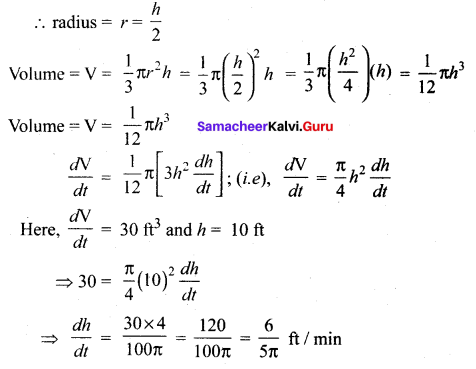So, the height is increasing at the rate of $$\frac{6}{5 \pi}$$ ft/min